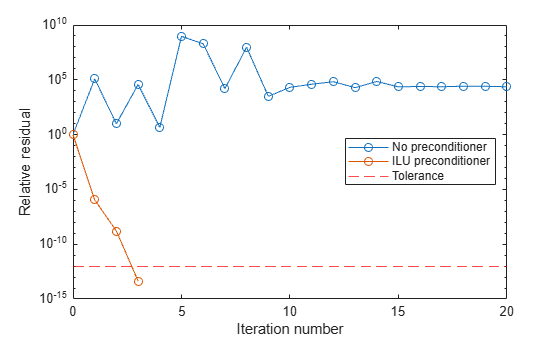Documentation

# cgs

## Syntax

```x = cgs(A,b) cgs(A,b,tol) cgs(A,b,tol,maxit) cgs(A,b,tol,maxit,M) cgs(A,b,tol,maxit,M1,M2) cgs(A,b,tol,maxit,M1,M2,x0) [x,flag] = cgs(A,b,...) [x,flag,relres] = cgs(A,b,...) [x,flag,relres,iter] = cgs(A,b,...) [x,flag,relres,iter,resvec] = cgs(A,b,...) ```

## Description

`x = cgs(A,b)` attempts to solve the system of linear equations `A*x = b` for `x`. The `n`-by-`n` coefficient matrix `A` must be square and should be large and sparse. The column vector `b` must have length `n`. You can specify `A` as a function handle, `afun`, such that `afun(x)` returns `A*x`.

Parameterizing Functions explains how to provide additional parameters to the function `afun`, as well as the preconditioner function `mfun` described below, if necessary.

If `cgs` converges, a message to that effect is displayed. If `cgs` fails to converge after the maximum number of iterations or halts for any reason, a warning message is printed displaying the relative residual `norm(b-A*x)/norm(b)` and the iteration number at which the method stopped or failed.

`cgs(A,b,tol)` specifies the tolerance of the method, `tol`. If `tol` is `[]`, then `cgs` uses the default, `1e-6`.

`cgs(A,b,tol,maxit)` specifies the maximum number of iterations, `maxit`. If `maxit` is `[]` then `cgs` uses the default, `min(n,20)`.

`cgs(A,b,tol,maxit,M)` and `cgs(A,b,tol,maxit,M1,M2)` use the preconditioner `M` or `M = M1*M2` and effectively solve the system ```inv(M)*A*x = inv(M)*b``` for `x`. If `M` is `[]` then `cgs` applies no preconditioner. `M` can be a function handle `mfun` such that `mfun(x)` returns `M\x`.

`cgs(A,b,tol,maxit,M1,M2,x0)` specifies the initial guess `x0`. If `x0` is `[]`, then `cgs` uses the default, an all-zero vector.

`[x,flag] = cgs(A,b,...)` returns a solution `x` and a flag that describes the convergence of `cgs`.

Flag

Convergence

`0`

`cgs` converged to the desired tolerance `tol` within `maxit`iterations.

`1`

`cgs` iterated `maxit` times but did not converge.

`2`

Preconditioner `M` was ill-conditioned.

`3`

`cgs` stagnated. (Two consecutive iterates were the same.)

`4`

One of the scalar quantities calculated during `cgs` became too small or too large to continue computing.

Whenever `flag` is not `0`, the solution `x` returned is that with minimal norm residual computed over all the iterations. No messages are displayed if the `flag` output is specified.

`[x,flag,relres] = cgs(A,b,...)` also returns the relative residual `norm(b-A*x)/norm(b)`. If `flag` is `0`, then ```relres <= tol```.

`[x,flag,relres,iter] = cgs(A,b,...)` also returns the iteration number at which `x` was computed, where `0 <= iter <= maxit`.

`[x,flag,relres,iter,resvec] = cgs(A,b,...)` also returns a vector of the residual norms at each iteration, including `norm(b-A*x0)`.

## Examples

### Using cgs with a Matrix Input

```A = gallery('wilk',21); b = sum(A,2); tol = 1e-12; maxit = 15; M1 = diag([10:-1:1 1 1:10]); x = cgs(A,b,tol,maxit,M1);```

displays the message

```cgs converged at iteration 13 to a solution with relative residual 2.4e-016.```

### Using cgs with a Function Handle

This example replaces the matrix `A` in the previous example with a handle to a matrix-vector product function `afun`, and the preconditioner `M1` with a handle to a backsolve function `mfun`. The example is contained in the file `run_cgs` that

• Calls `cgs` with the function handle `@afun` as its first argument.

• Contains `afun` as a nested function, so that all variables in `run_cgs` are available to `afun` and `myfun`.

The following shows the code for `run_cgs`:

```function x1 = run_cgs n = 21; b = afun(ones(n,1)); tol = 1e-12; maxit = 15; x1 = cgs(@afun,b,tol,maxit,@mfun); function y = afun(x) y = [0; x(1:n-1)] + ... [((n-1)/2:-1:0)'; (1:(n-1)/2)'].*x + ... [x(2:n); 0]; end function y = mfun(r) y = r ./ [((n-1)/2:-1:1)'; 1; (1:(n-1)/2)']; end end```

When you enter

`x1 = run_cgs`

MATLAB® software returns

```cgs converged at iteration 13 to a solution with relative residual 2.4e-016.```

### Using cgs with a Preconditioner.

This example demonstrates the use of a preconditioner.

Load `west0479`, a real 479-by-479 nonsymmetric sparse matrix.

```load west0479; A = west0479;```

Define `b` so that the true solution is a vector of all ones.

`b = full(sum(A,2));`

Set the tolerance and maximum number of iterations.

```tol = 1e-12; maxit = 20;```

Use `cgs` to find a solution at the requested tolerance and number of iterations.

`[x0,fl0,rr0,it0,rv0] = cgs(A,b,tol,maxit);`

`fl0` is 1 because `cgs` does not converge to the requested tolerance `1e-12` within the requested 20 iterations. In fact, the behavior of `cgs` is so poor that the initial guess (`x0 = zeros(size(A,2),1)` is the best solution and is returned as indicated by `it0 = 0`. MATLAB stores the residual history in `rv0`.

Plot the behavior of `cgs`.

```semilogy(0:maxit,rv0/norm(b),'-o'); xlabel('Iteration number'); ylabel('Relative residual');```The plot shows that the solution does not converge. You can use a preconditioner to improve the outcome.

Create a preconditioner with `ilu`, since `A` is nonsymmetric.

```[L,U] = ilu(A,struct('type','ilutp','droptol',1e-5)); ```
```Error using ilu There is a pivot equal to zero. Consider decreasing the drop tolerance or consider using the 'udiag' option. ```

MATLAB cannot construct the incomplete LU as it would result in a singular factor, which is useless as a preconditioner.

You can try again with a reduced drop tolerance, as indicated by the error message.

```[L,U] = ilu(A,struct('type','ilutp','droptol',1e-6)); [x1,fl1,rr1,it1,rv1] = cgs(A,b,tol,maxit,L,U);```

`fl1` is 0 because `cgs` drives the relative residual to `4.3851e-014` (the value of `rr1`). The relative residual is less than the prescribed tolerance of `1e-12` at the third iteration (the value of `it1`) when preconditioned by the incomplete LU factorization with a drop tolerance of `1e-6`. The output `rv1(1)` is `norm(b)` and the output `rv1(14)` is `norm(b-A*x2)`.

You can follow the progress of `cgs` by plotting the relative residuals at each iteration starting from the initial estimate (iterate number 0).

```semilogy(0:it1,rv1/norm(b),'-o'); xlabel('Iteration number'); ylabel('Relative residual');```## References

 Barrett, R., M. Berry, T. F. Chan, et al., Templates for the Solution of Linear Systems: Building Blocks for Iterative Methods, SIAM, Philadelphia, 1994.

 Sonneveld, Peter, “CGS: A fast Lanczos-type solver for nonsymmetric linear systems,” SIAM J. Sci. Stat. Comput., January 1989, Vol. 10, No. 1, pp. 36–52.